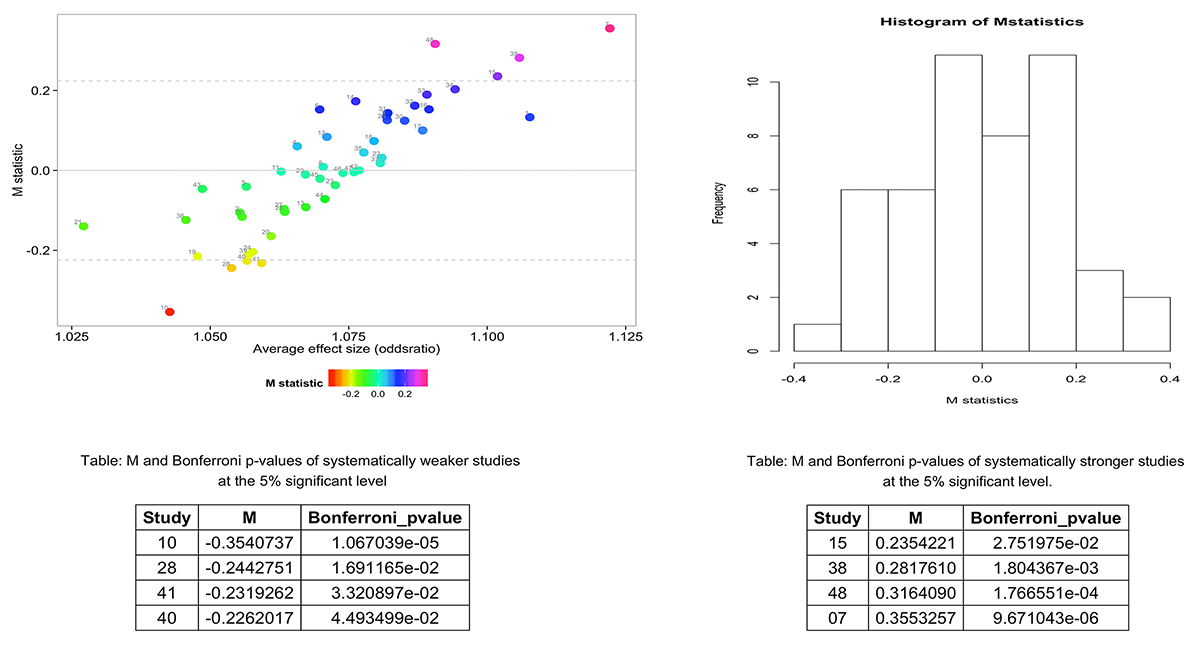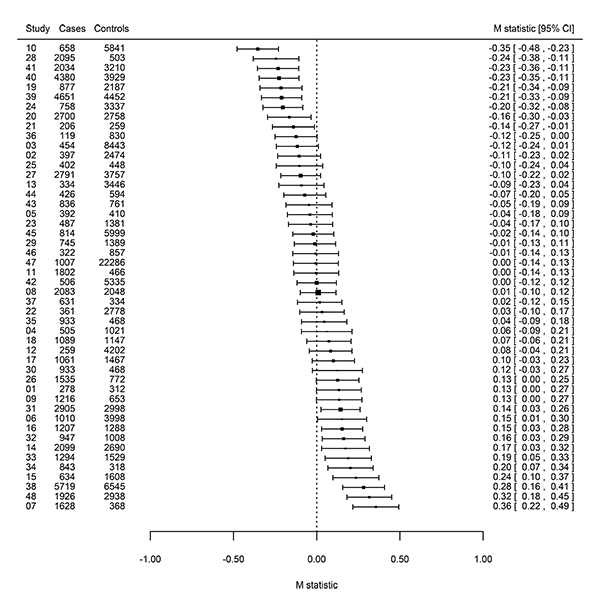## Background

• Statistical heterogeneity can be described as consistent study effect-size differences among participating studies in a meta-analysis, across the array of genetic variants examined.

• The getmstatistic function ?getmstatistic computes M statistics to quantitatively describe systematic heterogeneity in meta-analysis.

• M statistics are useful for identifying outlier studies which show null effects or consistently show stronger/weaker effects than average.

## Properties of the M-statistic

• M’s power to detect systematic heterogeneity patterns increases with the number and strength of association of variants examined in the meta-analysis.

• M statistics can be calculated using a panel of known lead variants independently associated with the trait of interest or the GWAS significant lead variants in a meta-analysis.

• Furthermore, M’s power is relatively indepenent of the number of studies in a meta-analysis.

• The M statistic can be applied to meta-analyses with few studies (e.g. 10 studies).

#### See the M statistic paper for details on the statistical theory:

Magosi LE, Goel A, Hopewell JC, Farrall M, on behalf of the CARDIoGRAMplusC4D Consortium (2017) Identifying systematic heterogeneity patterns in genetic association meta-analysis studies. PLoS Genet 13(5): e1006755. https://doi.org/10.1371/journal.pgen.1006755.

## A few tips for a succesful M analysis

Prior to conducting an M analysis:

• ensure that the variants that will be used to conduct the M analysis are independently associated with the trait of interest i.e. in linkage equilibrium.

• ensure that the effect alleles for participating studies in the meta-analysis are aligned. This can be achieved by “flipping” the beta-values (i.e. multiply by -1) of misaligned effect alleles.

• apply a study-level genomic corrrection to the standard errors to minimize false positives.

For example: gcse <- se * sqrt(lambda)

## Data: heartgenes214

#### We shall use the heartgenes214 dataset for our M analysis.


heartgenes214 is a multi-ethnic GWAS meta-analysis dataset for coronary
artery disease.

It comprises summary data (effect-sizes and their corresponding standard errors) for
48 studies (68,801 cases and 123,504 controls), at 214 lead variants independently
associated with coronary artery disease (P < 0.00005, FDR < 5%). Of the 214 lead variants,
44 are genome-wide significant (p < 5e-08). The meta-analysis dataset is based on individuals
of: African American, Hispanic American, East Asian, South Asian, Middle Eastern and European
ancestry.


#### Note:

• The beta-values in heartgenes214 have already been flipped to align effect alleles.

• Also, the standard errors have been genomically controlled at the study-level.

#### The data was sourced from:

Magosi LE, Goel A, Hopewell JC, Farrall M, on behalf of the CARDIoGRAMplusC4D Consortium (2017) Identifying systematic heterogeneity patterns in genetic association meta-analysis studies. PLoS Genet 13(5): e1006755. https://doi.org/10.1371/journal.pgen.1006755.

## The basics: Conducting an M analysis

The getmstatistic function requires the following variables for an M analysis:

• A numeric vector of beta-values i.e. study effect-sizes.
• A numeric vector of standard errors.
• A character vector of variant/SNP names.
• A character vector of study names.


library(getmstatistic)  # for calculating M statistics
library(gridExtra)       # for generating tables

# Basic M analysis using heartgenes214  --------------------------

# heartgenes214 is a multi-ethnic GWAS meta-analysis dataset for coronary artery disease.
# ?heartgenes214 to view the dataset documentation.

# View the structure of the heartgenes214 dataset
str(heartgenes214)

# Run M analysis on all 214 lead variants
# To view getmstatistic documentation ?getmstatistic or ?getm

getmstatistic_results <- getmstatistic(heartgenes214$beta_flipped, heartgenes214$gcse,
heartgenes214$variants, heartgenes214$studies)
getmstatistic_results

# Explore results generated by getmstatistic  --------------------

# Retrieve dataset of M statistics
dframe <- getmstatistic_results$M_dataset head(dframe) str(dframe) # Retrieve dataset of stronger than average studies (significant at 5% level) getmstatistic_results$influential_studies_0_05

# Retrieve dataset of weaker than average studies (significant at 5% level)
getmstatistic_results$weaker_studies_0_05 # Retrieve number of studies and variants getmstatistic_results$number_studies
getmstatistic_results$number_variants # Retrieve expected mean, sd and critical M value at 5% significance level getmstatistic_results$M_expected_mean
getmstatistic_results$M_expected_sd getmstatistic_results$M_crit_alpha_0_05



## Understanding the plots generated by getmstatisticM scatterplot: M statistics for each study in the meta-analysis (Y- axis) are plotted against the average variant effect size (expressed as odds ratios) (X-axis) in each study. The dashed lines indicate the Bonferroni corrected 5% significance threshold (M= ±0.483) to allow for multiple testing of 48 studies. Studies showing weaker (M < 0) than average genetic effects can be distinguished from those showing stronger (M > 0) than average effects.

## A step further: Exploring available options


# Run M analysis on GWAS significant lead variants  --------------------

# Subset the GWAS significant variants (p < 5e-08) in heartgenes214
heartgenes44 <- subset(heartgenes214, heartgenes214$fdr214_gwas46 == 2) # Exploring getmstatistic options: # Estimate heterogeneity using "REML", default is "DL" # Modify x-axis of M scatterplot # Run M analysis verbosely getmstatistic_results <- getmstatistic(heartgenes44$beta_flipped,
heartgenes44$gcse, heartgenes44$variants,
heartgenes44$studies, tau2_method = "REML", x_axis_increment_in = 0.03, x_axis_round_in = 3, verbose_output = TRUE) getmstatistic_results  ## Generating M statistic forest plots library(metafor) # for conducting meta-analysis library(dplyr) # for sorting data.frames # We shall use the results from the M analysis of the 214 lead variants in heartgenes214 # As a reminder, here is the command below: getmstatistic_results <- getmstatistic(heartgenes214$beta_flipped,
heartgenes214$gcse, heartgenes214$variants,
heartgenes214$studies) # Sort getmstatistic_results dataframe by M statistics getm_res_srtd <- dplyr::arrange(getmstatistic_results$M_dataset, M)

# Add on the case and control fields

# First, drop duplicate study_names in the sorted getmsatistic_results
getm_res_srtd_nodups <- subset(getm_res_srtd, getm_res_srtd$snp == 5) # Checking dimensions to confirm that we have 48 studies str(getm_res_srtd_nodups) # Second, drop duplicate study_names in heartgenes heartgenes214_nodups <- subset(heartgenes214, heartgenes214$variants == "chr14:75614504:I")
str(heartgenes214_nodups)

# Third, merge the two dataframes to add on the cases and controls
getm_res_plus_cases_ctrls <- merge(getm_res_srtd_nodups, heartgenes214_nodups[, c("studies", "cases", "controls")], by.x="study_names_in", by.y="studies")

# Sort data.frame by M statistics
getm_res_plus_cases_ctrls_srtd <- dplyr::arrange(getm_res_plus_cases_ctrls, M)

# Compute inverse-variance weighted fixed effects model
# using fixed effects model to get variable box size
metafor_results_fe <- metafor::rma.uni(yi = getm_res_plus_cases_ctrls_srtd[, "M"], sei = getm_res_plus_cases_ctrls_srtd[, "M_se"], weighted = T, slab = sprintf("%02.0f", getm_res_plus_cases_ctrls_srtd[, "study"]), method = "FE")
metafor_results_fe

# Compute inverse-variance weighted random effects model
metafor_results_dl <- metafor::rma.uni(yi = getm_res_plus_cases_ctrls_srtd[, "M"], sei = getm_res_plus_cases_ctrls_srtd[, "M_se"], weighted = T, knha = T, slab = sprintf("%2.0f", getm_res_plus_cases_ctrls_srtd[, "study"]), method = "DL")
metafor_results_dl

# Plotting:

# set margins
par(mar=c(4,4,1,2))

# generate forest plot
forest(metafor_results_fe, xlim=c(-1.8, 1.6), at=c(-1, -0.5, 0, 0.5, 1), cex=0.66, xlab = "M statistic", ilab=cbind(getm_res_plus_cases_ctrls_srtd$cases, getm_res_plus_cases_ctrls_srtd$controls), ilab.xpos = c(-1.4,-1.1), ilab.pos = c(2,2), addfit=F)